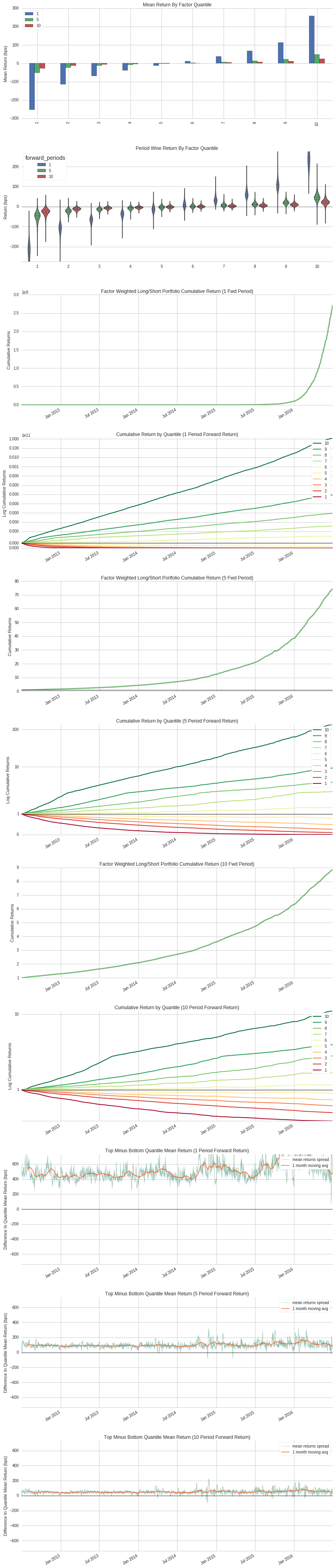Notebook

# Alphalens - Open Source Factor AnalysisÂ¶

In :
import numpy as np
import pandas as pd
import alphalens
from quantopian.research import run_pipeline
from quantopian.pipeline import Pipeline
from quantopian.pipeline.data.builtin import USEquityPricing
from quantopian.pipeline.data import morningstar
from quantopian.pipeline.factors import CustomFactor, Latest, Returns, RSI, EWMA as ewma, SimpleMovingAverage
from quantopian.pipeline.filters import Q1500US


## Define the FactorsÂ¶

In :
#Create the classes for many rav variables and factors

class LowVol(CustomFactor):
inputs = [Returns(window_length=2)]
window_length = 25

def compute(self, today, assets, out, close):
out[:] = -np.nanstd(close, axis=0)

class growth(CustomFactor):
window_length = 1
inputs = [morningstar.operation_ratios.revenue_growth]

def compute(self, today, assets, out, growth):
out[:] = growth

class sus_growth(CustomFactor):
window_length = 1
inputs = [morningstar.valuation_ratios.sustainable_growth_rate]

def compute(self, today, assets, out, sustainable_growth_rate):
out[:] = sustainable_growth_rate

#Quality
class turns(CustomFactor):
window_length = 1
inputs = [morningstar.operation_ratios.assets_turnover]

def compute(self, today, assets, out, assets_turnover):
out[:] = assets_turnover

class roic(CustomFactor):
window_length = 1
inputs = [morningstar.operation_ratios.roic]
def compute(self, today, assets, out, roic):
out[:] = roic

class margins(CustomFactor):
window_length = 1
inputs = [morningstar.operation_ratios.ebitda_margin]
def compute(self, today, assets, out, ebitda_margin):
out[:] = ebitda_margin

#Valuation
class peg_ratio(CustomFactor):
window_length = 1
inputs = [morningstar.valuation_ratios.peg_ratio]

def compute(self, today, assets, out, peg_ratio):
out[:] = np.where(peg_ratio <= 0, 0, 1/peg_ratio)

class pe_ratio(CustomFactor):
window_length = 1
inputs = [morningstar.valuation_ratios.pe_ratio]
def compute(self, today, assets, out, pe_ratio):
out[:] = np.where(pe_ratio <= 0, 0, 1/pe_ratio)

class pb_ratio(CustomFactor):
window_length = 1
inputs = [morningstar.valuation_ratios.pb_ratio]
def compute(self, today, assets, out, pb_ratio):
out[:] = np.where(pb_ratio <= 0, 0, 1/pb_ratio)

class total_yield(CustomFactor):
window_length = 1
inputs = [morningstar.valuation_ratios.total_yield]
def compute(self, today, assets, out, total_yield):
out[:] = total_yield

class ev_ebitda(CustomFactor):
window_length = 1
inputs = [morningstar.valuation_ratios.ev_to_ebitda]
def compute(self, today, assets, out, ev_to_ebitda):
out[:] = np.where(ev_to_ebitda <= 0, 0, 1/ev_to_ebitda)

class liquidity(CustomFactor):
inputs = [USEquityPricing.volume, USEquityPricing.close]
window_length = 21

def compute(self, today, assets, out, volume, close):
out[:] = (volume * close).mean(axis=0)

class sector(CustomFactor):
inputs = [morningstar.asset_classification.morningstar_sector_code]
window_length = 1
def compute(self, today, assets, out, morningstar_sector_code):
out[:] = morningstar_sector_code[-1]

inputs = [USEquityPricing.close, USEquityPricing.volume]
window_length = 20

def compute(self, today, assets, out, close_price, volume):
out[:] = np.mean(close_price * volume, axis=0)

class PriceReturn(CustomFactor):
inputs = [USEquityPricing.close]
window_length = 2

def compute(self, today, assets, out, close):
out[:] = close[-1]/close


## Set UniverseÂ¶

In :
universe = Q1500US()


## Create the PipelineÂ¶

In :
pipe = Pipeline(
columns={
},
screen=universe
)


## Run the PipelineÂ¶

In :
results = run_pipeline(pipe, '2012-06-30', '2016-06-30')

In :
results.head()

Out:
EV_EBITDA PriceReturn Sector
2012-07-02 00:00:00+00:00 Equity(2 [ARNC]) 0.067388 1.028235 101.0
Equity(24 [AAPL]) 0.099307 1.026795 311.0
Equity(52 [ABM]) 0.121308 0.985880 310.0
Equity(53 [ABMD]) 0.008095 1.041534 206.0
Equity(62 [ABT]) 0.171444 1.024622 206.0
In :
#Develop Factors for Testing
factor_name = 'EV_EBITDA'

results['factor'] = results.groupby([results.index.get_level_values(0),'Sector'])[factor_name].apply(lambda x: x.fillna(x.median()))

zscore = lambda x: (x - x.mean()) / x.std()
results['factor_score'] = results.groupby([results.index.get_level_values(0),'Sector'])['factor'].transform(zscore)

#Reset Factor to mean where abs(factor_score) > 3

results['forward_return_1'] = results.groupby(results.index.get_level_values(1))['PriceReturn'].apply(lambda x: x.shift(-1))


## Get pricingÂ¶

In :
assets = results.index.levels.unique()
# Make sure to extend the pricing a bit to get a full set of returns.
pricing = get_pricing(assets, start_date='2012-06-30', end_date='2016-07-31', fields='open_price')

In :
results.head()

Out:
2012-07-02 00:00:00+00:00 Equity(2 [ARNC]) 0.067388 1.028235 101.0 0.067388 -0.664975 0.067388 -0.664975 0.987414
Equity(24 [AAPL]) 0.099307 1.026795 311.0 0.099307 -0.090975 0.099307 -0.032519 1.014914
Equity(52 [ABM]) 0.121308 0.985880 310.0 0.121308 -0.082475 0.121308 -0.021833 0.992012
Equity(53 [ABMD]) 0.008095 1.041534 206.0 0.008095 -1.035993 0.008095 -1.186339 1.028046
Equity(62 [ABT]) 0.171444 1.024622 206.0 0.171444 0.901986 0.171444 1.221839 1.002172

## Build TearsheetÂ¶

AlphaLens builds a single factor tearsheet.

In :
#First, let's look at our standard factor
factor_data = alphalens.utils.get_clean_factor_and_forward_returns(results['EV_EBITDA'],
pricing,
quantiles=10,
periods=(1,5,10))

alphalens.tears.create_full_tear_sheet(factor_data)

Quantiles Statistics

min max mean std count count %
factor_quantile
1 0.115815 1.008523 0.969218 0.028089 150204 10.033386
2 0.919959 1.016150 0.987489 0.010797 149678 9.998250
3 0.933965 1.020658 0.992675 0.009579 149602 9.993173
4 0.944708 1.025122 0.996145 0.009031 149720 10.001055
5 0.951937 1.028907 0.998994 0.008774 149739 10.002325
6 0.959253 1.033234 1.001705 0.008692 149476 9.984757
7 0.964623 1.038395 1.004591 0.008810 149394 9.979279
8 0.968305 1.044556 1.008080 0.009186 149654 9.996647
9 0.973426 1.056765 1.013330 0.010129 149513 9.987228
10 0.981000 8.983420 1.032713 0.039007 150062 10.023900
Returns Analysis

1 5 10
Ann. alpha 235.079 1.963 0.734
beta -0.058 -0.037 -0.028
Mean Period Wise Return Bottom Quantile (bps) -252.796 -254.882 -262.501
Mean Period Wise Spread (bps) 510.936 100.644 50.926
Information Analysis

1 5 10
IC Mean 0.822 0.339 0.236
IC Std. 0.059 0.104 0.107
t-stat(IC) 437.814 103.106 70.102
p-value(IC) 0.000 0.000 0.000
IC Skew -3.815 -0.466 -0.320
IC Kurtosis 40.775 1.836 1.058
Ann. IR 219.233 51.630 35.103
Turnover Analysis

1 5 10
Quantile 1 Mean Turnover 0.825 0.836 0.840
Quantile 2 Mean Turnover 0.896 0.896 0.895
Quantile 3 Mean Turnover 0.896 0.897 0.897
Quantile 4 Mean Turnover 0.889 0.892 0.893
Quantile 5 Mean Turnover 0.881 0.887 0.888
Quantile 6 Mean Turnover 0.884 0.887 0.888
Quantile 7 Mean Turnover 0.890 0.891 0.891
Quantile 8 Mean Turnover 0.896 0.896 0.897
Quantile 9 Mean Turnover 0.896 0.896 0.898
Quantile 10 Mean Turnover 0.841 0.852 0.854
1 5 10
Mean Factor Rank Autocorrelation -0.008 0.001 0.005
<matplotlib.figure.Figure at 0x7f2e38a96450>